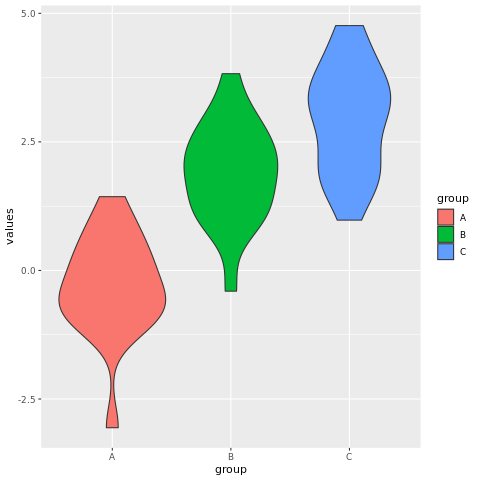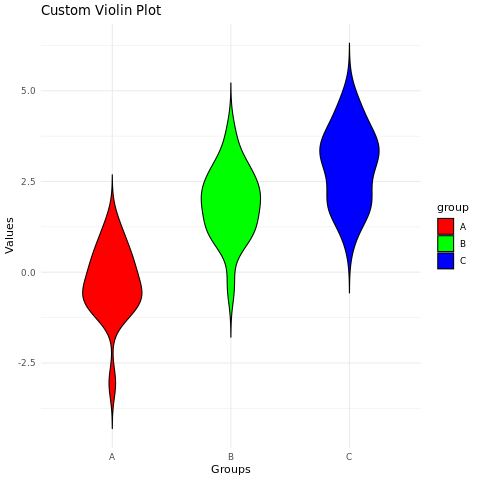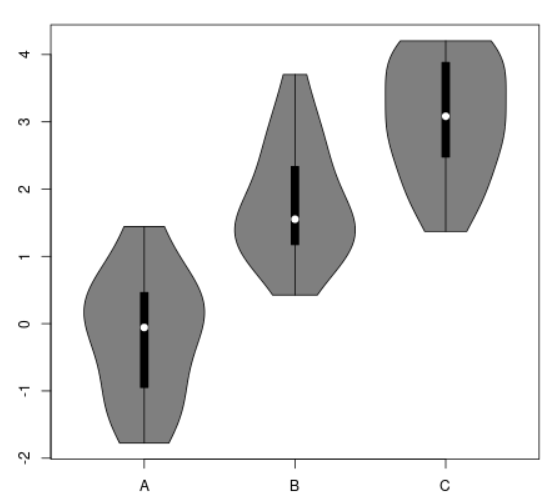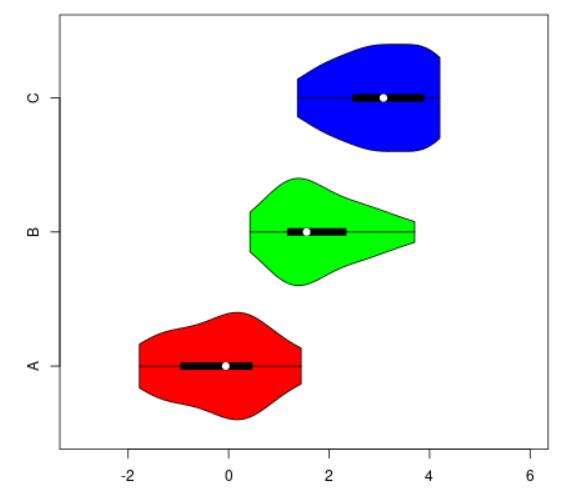# Violin Chart in R

A violin plot is a data visualization that combines aspects of a box plot and a density plot. It provides insights into the distribution of a continuous variable across different categories or groups. The violin plot shows the distribution of a continuous variable, including the median, quartiles, and outliers, as well as the density of the distribution.

In R, violin plots can be created using the geom_violin() function from the ggplot2 package. The syntax is as follows:

ggplot(data = data, aes(x = variable, y = value)) + geom_violin()
1. data is the data frame that contains the data.
2. variable is the name of the variable that is plotted on the x-axis.
3. value is the name of the variable that is plotted on the y-axis.

For example, the following code creates a violin plot of the height variable:

ggplot(data = iris, aes(x = Species, y = Sepal.Length)) + geom_violin()

This will create a violin plot with the Species variable plotted on the x-axis and the Sepal.Length variable plotted on the y-axis. The violin plots will show the distribution of the sepal length for each species of iris.

## geom_violin() function

The geom_violin() function has many options that can be used to customize the appearance of the violin plot. These options can be used to change the colors of the violins, the line styles, and the transparency of the violins.

For example, the following code changes the colors of the violins to red and green:

ggplot(data = iris, aes(x = Species, y = Sepal.Length)) + geom_violin(col = c("red", "green"))
1. The col option specifies the colors of the violins.

In R, you can create violin plots using various packages, with ggplot2 and the vioplot package being common choices.

## Violin Plot in R using ggplot2 Package

### Install and Load Required Packages

install.packages("ggplot2") library(ggplot2)

### Create a Violin Plot using ggplot2

To create a violin plot using ggplot2, you can use the geom_violin() function.

# Example: Creating a violin plot using ggplot2 data <- data.frame( group = rep(c("A", "B", "C"), each = 20), values = c(rnorm(20), rnorm(20, mean = 2), rnorm(20, mean = 3)) ) ggplot(data, aes(x = group, y = values, fill = group)) + geom_violin()

In this example, the group variable represents the categorical groups on the x-axis, and the values variable represents the continuous values on the y-axis.

### Customize the Violin Plot

You can customize the violin plot by adjusting parameters, adding labels, modifying colors, and more.

# Example: Customizing the violin plot using ggplot2 ggplot(data, aes(x = group, y = values, fill = group)) + geom_violin(trim = FALSE, scale = "width", width = 0.5, color = "black") + labs(title = "Custom Violin Plot", x = "Groups", y = "Values") + scale_fill_manual(values = c("A" = "red", "B" = "green", "C" = "blue")) + theme_minimal()

In this example, the trim parameter is used to control whether the density tails extend beyond the data range. The scale parameter determines whether the violin widths are scaled equally or based on the data. The width parameter sets the width of the violins. The scale_fill_manual() function is used to set custom fill colors for each group.

### Full Source | R

# Install and load the ggplot2 package install.packages("ggplot2") library(ggplot2) # Example: Creating a violin plot using ggplot2 data <- data.frame( group = rep(c("A", "B", "C"), each = 20), values = c(rnorm(20), rnorm(20, mean = 2), rnorm(20, mean = 3)) ) # Create the violin plot ggplot(data, aes(x = group, y = values, fill = group)) + geom_violin() # Example: Customizing the violin plot using ggplot2 ggplot(data, aes(x = group, y = values, fill = group)) + geom_violin(trim = FALSE, scale = "width", width = 0.5, color = "black") + labs(title = "Custom Violin Plot", x = "Groups", y = "Values") + scale_fill_manual(values = c("A" = "red", "B" = "green", "C" = "blue")) + theme_minimal()

### Output:## Violin Plot in R using vioplot Package

### Install and Load Required Packages

install.packages("vioplot") library(vioplot)

### Create a Violin Plot using vioplot

To create a violin plot using the vioplot package, you can use the vioplot() function.

# Example: Creating a violin plot using vioplot data <- list( A = rnorm(20), B = rnorm(20, mean = 2), C = rnorm(20, mean = 3) ) vioplot(data, names = c("A", "B", "C"))

In this example, the data list contains multiple datasets corresponding to different groups.

### Customize the Violin Plot

You can customize the violin plot using various parameters provided by the vioplot() function.

# Example: Customizing the violin plot using vioplot vioplot(data, names = c("A", "B", "C"), col = c("red", "green", "blue"), horizontal = TRUE, drawRect = TRUE, ylim = c(-3, 6))

In this example, the col parameter is used to set custom colors for each violin. The horizontal parameter is set to TRUE to create horizontal violins. The drawRect parameter is set to TRUE to add rectangles for each distribution. The ylim parameter is used to customize the y-axis limits.

### Full Source | R

# Install and load the vioplot package install.packages("vioplot") library(vioplot) # Example: Creating a violin plot using vioplot data <- list( A = rnorm(20), B = rnorm(20, mean = 2), C = rnorm(20, mean = 3) ) # Create the violin plot vioplot(data, names = c("A", "B", "C")) # Example: Customizing the violin plot using vioplot vioplot(data, names = c("A", "B", "C"), col = c("red", "green", "blue"), horizontal = TRUE, drawRect = TRUE, ylim = c(-3, 6))

### Output:### Conclusion

Violin plots in R can be created using the ggplot2 package or the vioplot package. They provide insights into the distribution of data within different groups and allow for customization to enhance visualization. By adjusting parameters, modifying colors, and comparing multiple datasets, you can create informative and visually appealing violin plots for data analysis and presentation.